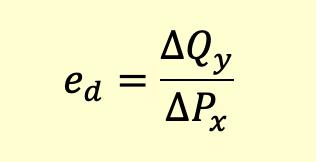# Cross Price Elasticity of Demand

Cross price elasticity of demand refers to the responsiveness of demand of one good to changes in the price of a related good (either a substitute or a complementary product).The above equation calculates the price elasticity of demand for good y for a change in price of good x.

For complementary products, cross price elasticity will have negative sign, signifying an inverse relationship between the two. So, when the price of a complementary product rises, the demand for the product itself decreases.

For a substitute, cross price elasticity will have a positive sign, signifying a positive relationship between the two. So, when the price of a substitute product rises, the demand for the product itself increases.